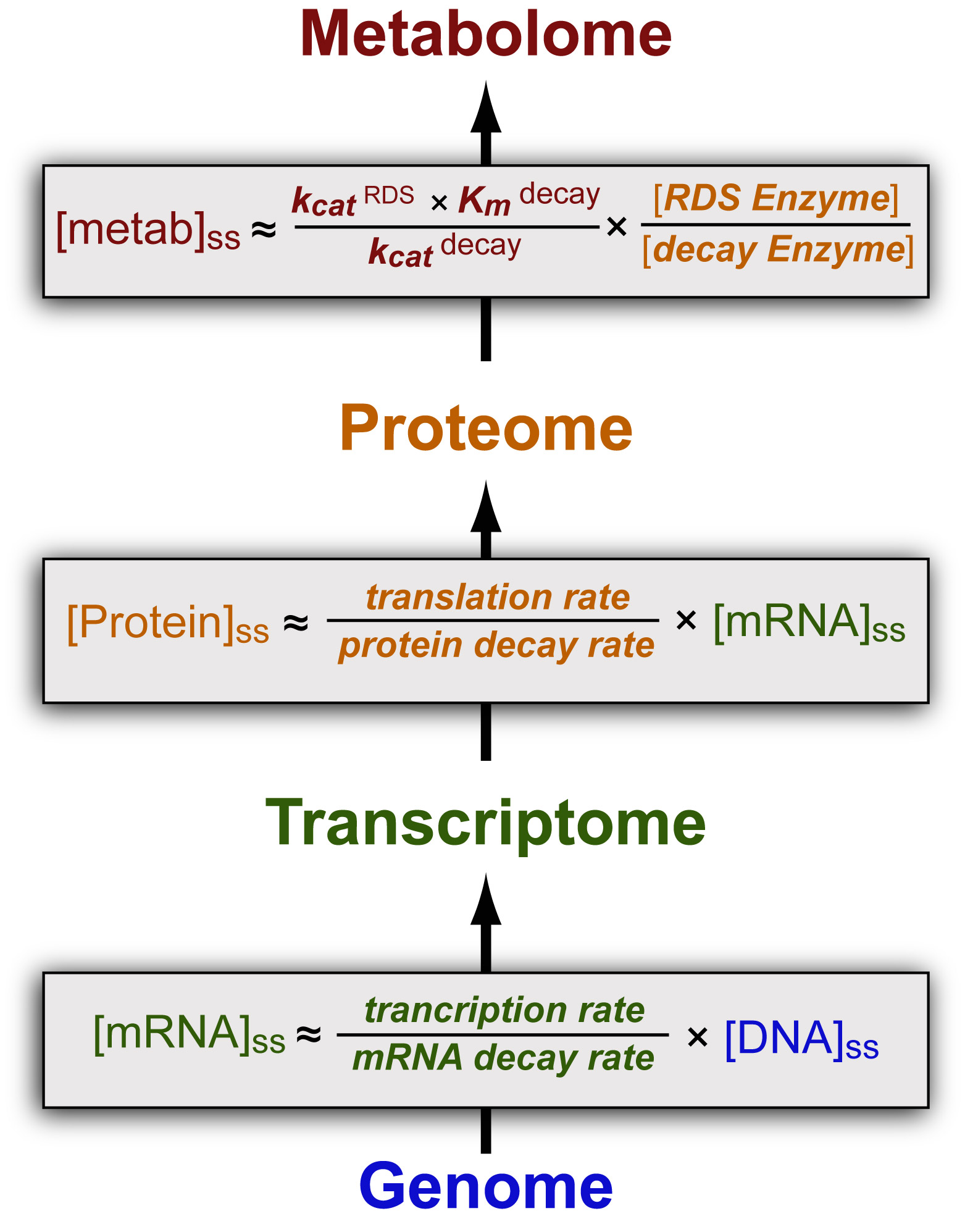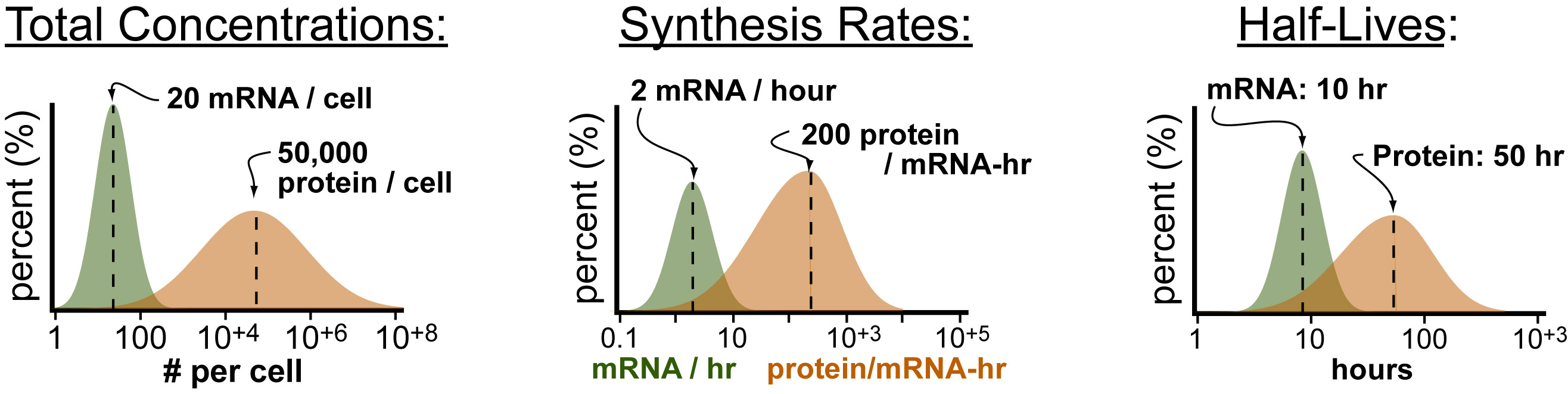# Estimating Metabolite/Protein Concentrations from RNAseq DataUnder steady-state conditions, it is possible to estimate the concentration of a metabolite from the amount of protein and the amount of protein from the amount of mRNA (see figure above). In general, the conversion factors used for these calculations are simply the ratio of the “first-order” formation and degredation rate constants for the protein/metabolite of interest. Recently, a paper published in Nature, characterized the distribution per gene of: (1) total mRNA and protein (2) rates of mRNA and Protein synthesis and (3) rates of mRNA and protein degradation (see figure below).Understanding mRNA and Protein levels:

As presented, the synthesis rates of mRNA are in “mRNA per hour” which is equivalent to “transcription rate x [DNA]ss.” Given the fact that the mRNA half-live is approximately the recipricol of the mRNA-decay rate constant, you can estimate the concentration of mRNA by multiplying “2 mRNA/hour” by “10 hours” which gives you approximately 20 mRNA (per gene) per cell which is very close to the median mRNA actually measured!

Similarly, if you multiply the median translation rate (“200 protein per mRNA per hour”) by the median mRNA concentration (“20 mRNA”) by the median protein half-life (“50 hours”) you can estimate the average total concentration of protein (per gene) at 200,000 per cell. While this does differ from the measured median, “50,000 protein (per gene) per cell”, it does give you an estimate within an order of magnitude.

Understanding Metabolite Level:

The conversion factor from enzyme concentrations to metabolite concentrations is a bit different because each enzyme utilized totally different chemistry (and thus rates) to make or degrade a molecule of interest. In general this breadth of chemistry is reflected in the breath of dissociation or Michaelis-Menton constants (Kd or Km) ranging from 100nM to 100uM and a range of kcat (inverse of turn over number) from 0.01s-1 to 1s-1. In general the rate of formation of a metabolite is determined by the enzyme that catalyzes the rate determining step (RDS) in the pathway that precedes it and the rate of decay is determined by the enzyme that directly degrades it.

REFERENCES: